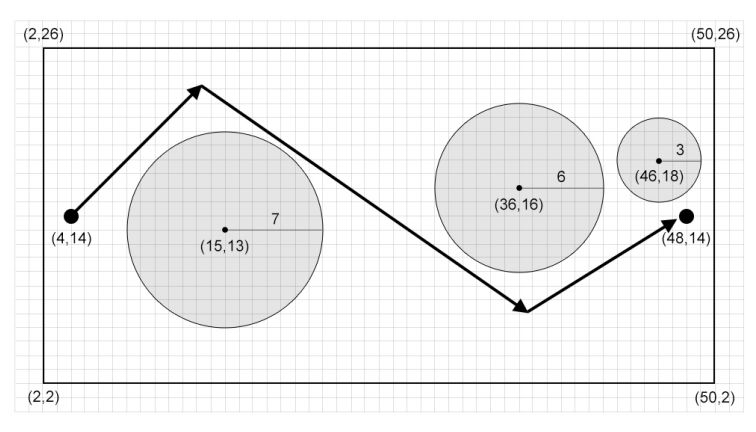시간 제한 메모리 제한 제출 정답 맞은 사람 정답 비율
1 초 512 MB 1 1 1 100.000%

## 문제

Allen, a government secret service, has been assigned to infiltrate a mafia secret base to uncover crucial information regarding the mafia’s operations.

The secret base is a rectangular bounded by (xL, yL), (xL, yR), (xR, yL), and (xR, yR) in a Cartesian coordinate system where xL < xR and yL < yR. There are N sensors placed inside the secret base. The ith sensor is located at (xi, yi) and has an effective sensing radius of ri which can detect any person who is strictly within the radius of ri from (xi, yi). In other words, the ith sensor can detect a person at location (xa, ya) if and only if the Euclidean distance of (xi, yi) and (xa, ya) is strictly less than ri. It is also known that the Euclidean distance of any two sensors i and j is strictly larger than ri + rj. Note that the Euclidean distance of two points, (xa, ya) and (xb, yb), is √(|xa − xb|2 + |ya − yb|2).

Allen begins his infiltration mission at location (xs, ys), and his target is located at (xt, yt). Allen has the power to run extremely fast in a straight line while he needs to spend extra time to change his running trajectory (to adjust his footing). Although he is a fast runner, he still needs to make sure that none of the sensors detect him while he is running, i.e. there is no point in his running trajectory which is strictly within a sensor effective sensing radius.

Let P = {(xp1, yp1), . . . ,(xp|P|, yp|P|)} be the set of locations where Allen changes his running trajectory, thus, Allen’s running trajectory with P is (xs, ys) → (xp1, yp1) → · · · → (xp|P|, yp|P|) → (xt, yt) where (xa, ya) → (xb, yb) implies that Allen is running from (xa, ya) to (xb, yb) in a straight line. The set P is feasible if and only if with P, Allen is not detected by any sensor and is not running out of the secret base (although, Allen is allowed to run along the secret base perimeter). Note that xp and yp, (xp, yp) ∈ P, are not necessarily integers; they can be real numbers.

Your task in this problem is to find any one feasible P which contains no more than 1000 points.

## 입력

Input begins with a line containing five integers: N xL yL xR yR (0 ≤ N ≤ 50; 0 ≤ xL < xR ≤ 1000; 0 ≤ yL < yR ≤ 1000) representing the number of sensors and the secret base (xL, yL, xR, yR), respectively. The next line contains two integers: xs ys (xL < xs < xR; yL < ys < yR) representing Allen’s initial location. The next line contains two integers: xt yt (xL < xt < xR; yL < yt < yR) representing Allen’s target location. It is guaranteed that xs ≠ xt or ys ≠ yt. The next N lines each contains three integers: xi yi ri (xL < xi − ri < xi + ri < xR; yL < yi − ri < yi + ri < yR; 1 ≤ ri ≤ 1000) representing a sensor at location (xi, yi) with an effective sensing radius of ri. It is guaranteed that the Euclidean distance of any two sensors i and j is larger than ri + rj. It is also guaranteed that the Euclidean distance of (xs, ys) and (xt, yt) to any sensor i is larger than ri.

## 출력

Output in a line an integer representing the size of a feasible P. The next |P| lines each contains two real numbers (separated by a single space); the jth line contains xj yj representing the jth point in P. You may output any feasible P with no more than 1000 points.

Due to the nature of the output (floating point), let us define an epsilon ε to be 10−6 to verify the output. Consider Q1 = (xs, ys), Qj+1 = Pj for all 1 ≤ j ≤ |P|, and Q|P|+2 = (xt, yt). Then, P is considered correct if and only if P contains no more than 1000 points and all of the following are satisfied:

• xL − ε ≤ xpk ≤ xR + ε and yL − ε ≤ ypk ≤ yR + ε for all 1 ≤ k ≤ |P| (Allen is not running out of the secret base).
• For all 1 ≤ k < |Q|, let Sk be the line segment connecting Qk and Qk+1 (Allen is running in straight line). For all 1 ≤ i ≤ N, let (xk,i, yk,i) be the point along Sk that is the closest to the ith sensor’s location, (xi, yi). Let dk,i be the Euclidean distance between (xk,i, yk,i) and (xi, yi). Then, the constraint ri ≤ dk,i + ε should be satisfied (Allen is not detected by any sensor).
• All points in Q are distinct. Two points, (xa, ya) and (xb, yb), are considered distinct if and only if |xa − xb| > ε or |ya − yb| > ε.

## 예제 입력 1

3 2 2 50 26
4 14
48 14
15 13 7
36 16 6
46 18 3


## 예제 출력 1

2
13.25 23.1234567
36.591003 7.1The figure above shows the P from the sample output. Note that there exists a feasible P with only one point in this sample, although you are not required to find such P.

## 예제 입력 2

1 0 0 1000 1000
100 501
900 501
500 251 250


## 예제 출력 2

0